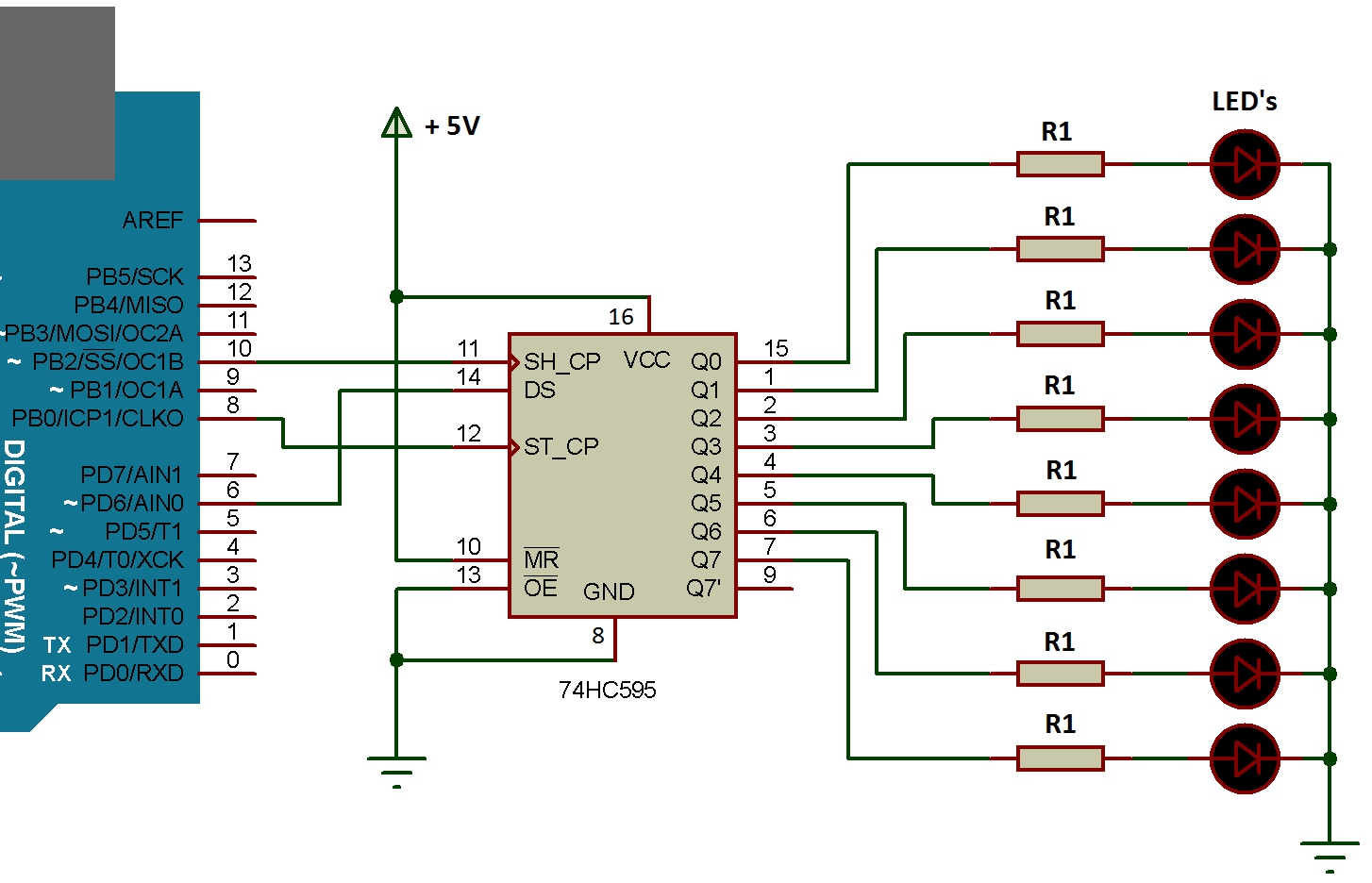# Shift Register IC 74HC595

Sometimes we have a number of modules to connect over our arduino, but Arduino UNO have 14 digital I/O pins, to avoid this problem we can use shift register IC.

Shift register IC is like a 3 input and 8 pin digital output decoder, where we use three pins of arduino for our signal that will be fed to three input lines of IC and from the output of IC we can decode it back into 8 output lines or bits.## SHIFT REGISTER IC 74HC595 PIN CONFIGURATION

Pin NumberPin NameDescription
15, 1, 2, 3, 4, 5, 6, 7Output Pins (Q0 to Q7)The 74hc595 has 8 output pins.
8GroundConnected to the Ground  of the circuit
9(Q7') Serial OutputThis pin is used to connect more than one 74hc595 as cascading (Pin 9 is data out and used when we have to connect another shift register, Feed this pin to next Shift register IC, if connected)
10(MR) Master ResetResets all outputs as low. Must be held high for normal operation
11(SH_CP) ClockThis is the clock pin to which the clock signal has to be provided from MCU/MPU
12(ST_CP) LatchThe Latch pin is used to update the data to the output pins.
13(OE) Output EnableThe Output Enable is used to turn off the outputs. Must be held low for normal operation
14(DS) Serial DataThis is the pin to which data is sent, based on which the 8 outputs are controlled
16VccThis pin powers the IC, typically +5V is used.

## SHIFT REGISTER IC 74HC595

How Shift Register IC works? We send 1 byte (8 bits) on the data pin (DS, pin 14) from our Arduino say 11000001, then you will see 3 pins of IC are HIGH and & 5 pins are LOW. (Here 1 represents HIGH & 0 represents LOW)

Now the question arises. Which pins are to be HIGH and which to be LOW?

As the bits represent data of byte variable (showing logics HIGH and LOW), and are responsible for output pins state in an order.

### DATA TRANSFER SEQUENCE

Transmitted: 1st Bit → Q7

Transmitted: 8th Bit (Last Bit) → Q0

We can send data in two modes

1. MSB First
2. LSB First (See Image below)Suppose, if the byte signal is B11001100 and we used MSB first, then the output of pins are

 Decimal Q7 Q6 Q5 Q4 Q3 Q2 Q1 Q0 204 1 1 0 0 1 1 0 0 2 – MSB, 4 – LSB MSB LSB

till the next cycle of the Byte. But we can change the orientation of MSB & LSB through programming we will see next.

Now, let’s connect 8 led to shift register IC to display 0 – 255 binary numbers pattern and to control them using only 3 data pins (digital) of the Arduino.

### CIRCUIT DIAGRAM### LED BINARY NUMBER DISPLAY

In this, we will pass a number of “decimal number system” and display its binary in the form of LED. For example, the number is 14 & its 8-bit binary equivalent is B00001110 then we will see first 4 led’s OFF, then 3 led’s ON and then last led OFF as well.

We know the highest 8-bit binary number is B11111111 and its equivalent decimal number is 255. So we are going to display all numbers turn by turn from 0 to 255.

After making this circuit upload the sketch given below:

### PROGRAMMING CODE

```// Creating an LED Binary Number Display
#define DATA 6      // digital 6 to pin 14 on the 74HC595
#define LATCH 8     // digital 8 to pin 12 on the 74HC595
#define CLOCK 10    // digital 10 to pin 11 on the 74HC595
int i = 0;
void setup() {
pinMode(LATCH, OUTPUT);
pinMode(CLOCK, OUTPUT);
pinMode(DATA, OUTPUT);
}
void loop() {
for ( i = 0; i < 256; i++ ) {
digitalWrite(LATCH, LOW);
shiftOut(DATA, CLOCK, MSBFIRST, i);
digitalWrite(LATCH, HIGH);
delay(200);
}
}
```

### CODE EXPLANATION

##### DEFINE VARIABLE

First of all, we are defining three pins DATA, LATCH & CLOCK as 6, 8 & 10 respectively and a variable i of int data type and initialize as 0.

##### SETUP

We simply set all three pins as OUTPUT, main logic is inside the loop

##### LOOP

1. A for loop runs from 0 to 255 for i

2. Then LATCH pin is set to LOW

3. Then using a function shiftOut()

In shiftOut() function we have to pass 4 arguments of integer type, 1st one data pin i.e. from which arduino pin data to transmit over shift register IC, 2nd which pin is used as clock, 3rd interrupt direction, then 4th what is data, here is ‘i’ the data (number from 0 to 255).

We send a decimal number from arduino to shift register IC in binary form, for example 240 as B11110000 from arduino digital pin 6 and tells the shiftOut() function from which direction to interrupt the signal byte either LSB or MSB

a) If LSBFIRST selected, then D7 (bit) denotes the Q0 (pin of shift register IC)

b) If MSBFIRST selected, then D7 (bit) denotes the Q7 (pin of shift register IC)

4. Then finally set the Latch pin as HIGH, this tells the shift register that bits are shifted and ready, at this point it vanishes previous output and updates new data.

5. End the cycles repeats every half second or 500 millisecond

6. After completion of for loop again loop starts from the beginning i.e. from 0 to 255.

SEVEN SEGMENT LED TYPES AND PIN CONFIG

5 1 vote
Article Rating
Subscribe
Notify of